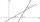# Angle + cosine - math problems

#### Number of problems found: 123

• CosineThe point (3, 4) is on the terminal side of angle θ. cos θ = ?
• CosineCalculate the cosine of the smallest internal angle in a right-angled triangle with cathetus 3 and 8 and with the hypotenuse 8.544.
• Right triangle trigonometricsCalculate the size of the remaining sides and angles of a right triangle ABC if it is given: b = 10 cm; c = 20 cm; angle alpha = 60° and the angle beta = 30° (use the Pythagorean theorem and functions sine, cosine, tangent, cotangent)
• Angle between linesCalculate the angle between these two lines: ? ?
• ABCDAC= 40cm , angle DAB=38 , angle DCB=58 , angle DBC=90 , DB is perpendicular on AC , find BD and AD
• BuildingThe building I focused at an angle 30°. When I moved 5 m building I focused at an angle 45°. What is the height of the building?
• Scalar dot productCalculate u.v if |u| = 5, |v| = 2 and when angle between the vectors u, v is: a) 60° b) 45° c) 120°
• Scalene triangleSolve the triangle: A = 50°, b = 13, c = 6
• Greatest angleCalculate the greatest triangle angle with sides 197, 208, 299.
• CuboidDetermine the dimensions of cuboid a, b, c; if diagonal d=9 dm has angle with edge a α=55° and has angle with edge b β=58°
• Angle between vectorsFind the angle between the given vectors to the nearest tenth of a degree. u = (-22, 11) and v = (16, 20)
• MastMast has 13 m long shadow on a slope rising from the mast foot in the direction of the shadow angle at angle 13.3°. Determine the height of the mast, if the sun above the horizon is at angle 45°12'.
• CotangentIf the angle α is acute, and cotg α = 1/3. Determine the value of sin α, cos α, tg α.
• CuboidsTwo separate cuboids with different orientation in space. Determine the angle between them, knowing the direction cosine matrix for each separate cuboid. u1=(0.62955056, 0.094432584, 0.77119944) u2=(0.14484653, 0.9208101, 0.36211633)
• DiagonalsCalculate the length of the diagonals of the rhombus if its side is long 5 and one of its internal angle is 80°.
• Right angleIn a right triangle ABC with a right angle at the apex C, we know the side length AB = 24 cm and the angle at the vertex B = 71°. Calculate the length of the legs of the triangle.
• TreeBetween points A and B is 50m. From A we see a tree at an angle 18°. From point B we see the tree in three times bigger angle. How tall is a tree?
• CloudsApproximately at what height is the cloud we see under an angle of 26°10' and see the Sun at an angle of 29°15' and the shade of the cloud is 92 meters away from us?
• Side cIn △ABC a=2, b=4 and ∠C=100°. Calculate length of the side c.
• TrigonometryIf you know that cos(γ) = sin (806°), what is the angle γ?

Do you have an interesting mathematical word problem that you can't solve it? Submit a math problem, and we can try to solve it.

We will send a solution to your e-mail address. Solved examples are also published here. Please enter the e-mail correctly and check whether you don't have a full mailbox.

Please do not submit problems from current active competitions such as Mathematical Olympiad, correspondence seminars etc...

Angle Problems. Cosine - math word problems.